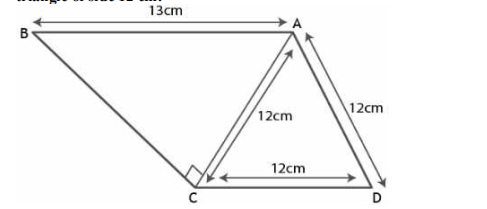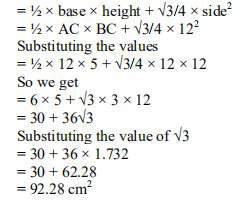Ml aggarwal solutionsOur top 5% students will be awarded a special scholarship to Lido.
Set your child up for success with Lido, book a class today!

# Question 1

. Find the area of the quadrilateral ABCD in which ∠BCA =90^{\circ}, AB = 13 cm and ACD is an equilateral

triangle of side 12 cm.• Solution

• Transcript

It is given that

ABCD is a quadrilateral in which ∠BCA =90^{\circ} and AB = 13 cm

ABCD is an equilateral triangle in which AC = CD = AD = 12 cm

In right-angled △ABC

Using Pythagoras theorem,

\begin{aligned} &\mathrm{AB}^{2}=\mathrm{AC}^{2}+\mathrm{BC}^{2}\\ &\text { Substituting the values } \end{aligned}

\begin{aligned} &13^{2}=12^{2}+\mathrm{BC}^{2}\\ &\text { By further calculation }\\ &\mathrm{BC}^{2}=13^{2}-12^{2}\\ &\mathrm{BC}^{2}=169-144=25 \end{aligned}

So we get

BC = √25 = 5 cm

We know that

Area of quadrilateral ABCD = Area of △ABC + Area of △ACD

It can be written as"hello and welcome my dear students i am rishabh the math uternal leader and i'm here the new question it is find the area of the quadrilateral abcd in which angle bca is 90 degrees a b is 13 and a c d is an equivalent triangular side 12 centimeter so without wasting much time let's have a look at the solution we know that abcd is a coordinator in which angle bca is 90 degree and angle a b is and a b is given as 13 centimeter also the acd is in triangle acd is an equilibrium triangle i'm writing eq for equilibrium of an edge 12 centimeter so in triangle abc because we know that triangle abc is a right angle triangle so we'll apply the pythagoras theorem so using pi is theorem you say b a b square is equals to ac square plus bc square so if i substitute the values we get bc square as 13 square minus 12 square which is nothing but equals to 169 minus 144 which is nothing but equals 25 which further implies that vc is equals to 20 by bc reverse to 5. now to calculate the area of the quadrilateral abcd this is equals to area of triangle abc plus area of triangle act which is nothing but equals to half into base into height plus root 3 by 4 into side square summating s plus i for the substituting the values we get half into ac into bc plus root 3 into 12 square so ac is 5 ac is 12 and bc is 5 plus root 3 into 3 into 12 that means solving it we get 6 into 5 plus 36 root 3 which is equals to 30 plus 36 into 1.73 2 which is the value of root 3 so solving it further we get 92.28 centimeter square as the area so i hope my students got this question well do not forget to hit the subscribe button you can also share your doubts in the comment section see you next time bye"

Our top 5% students will be awarded a special scholarship to Lido.Lido

Courses

Teachers

Book a Demo with us

Syllabus

Science
English
Coding

Terms & Policies

NCERT Question Bank

Maths
Science

Selina Question Bank

Maths
Physics
Biology

Allied Question Bank

Chemistry